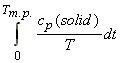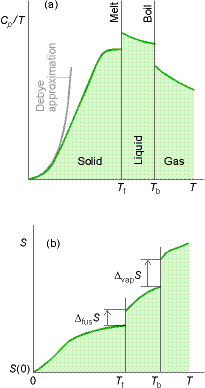Back to Table of Contents [TOP]   The third law of thermodynamics (Nerst heat theorem) and absolute entropies   The third law of thermodynamics (Nerst heat theorem) staes that the energy of a perfect crystal at 0K is 0.  Note - This perfection can never be reached (e.g. isotopes, different nuclear spins) and gives rise to the so called residual entropy.  Standard/Absolute entropies Def'n: The standard entropyof a compound is the molar entropy at 298.15K and 1 Bar pressure.    The entropy at a temperature T (and 1 Bar pressure) is calculated as follows (see figure below) :(1) All the properties (except S(0) can be measured experimentally. Note that if we assume that S(0) = 0, then the entropy takes the name of absolute (or third law) entropy. (2) The integrals can be either calculated graphically from the curve 'cp/T' vs. 'T' (see fig. below), by fitting a polynomial and then integrating the polynomial analytically, or by evaluating the area of a plot of cp vs ln(T).  (3) T here is a problem in evaluating the integral:since it is difficult to evaluate cp in the limit of 0K. We hence make use of the Debye extrapolation, i.e. (a) measure cp to as low a temperature as possible; (b) fit a curve of cp = aT3 to the data collected, and determine the value of 'a'; (c) Assume that the expression cp = aT3 remains valid up to T = 0K.Fig. 1: The determination of entropy from experimental data